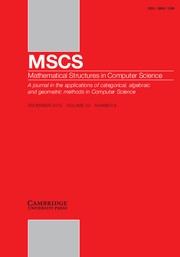Home
Hostname: page-component-5c569c448b-bmzkg Total loading time: 0.27 Render date: 2022-07-01T13:57:32.658Z Has data issue: true Feature Flags: { "shouldUseShareProductTool": true, "shouldUseHypothesis": true, "isUnsiloEnabled": true, "useRatesEcommerce": false, "useNewApi": true } hasContentIssue trueMathematical Structures in Computer Science

# Two-stage submodular maximization problem beyond nonnegative and monotone

Published online by Cambridge University Press:  16 November 2021

*
*Corresponding author. Emails: royxyzhang@gmail.com; zhangxiaoyan@njnu.edu.cn

## Abstract

We consider a two-stage submodular maximization problem subject to a cardinality constraint and k matroid constraints, where the objective function is the expected difference of a nonnegative monotone submodular function and a nonnegative monotone modular function. We give two bi-factor approximation algorithms for this problem. The first is a deterministic$\left( {{1 \over {k + 1}}\left( {1 - {1 \over {{e^{k + 1}}}}} \right),1} \right)$-approximation algorithm, and the second is a randomized$\left( {{1 \over {k + 1}}\left( {1 - {1 \over {{e^{k + 1}}}}} \right) - \varepsilon ,1} \right)$-approximation algorithm with improved time efficiency.

## Keywords

Type
Special Issue: Theory and Applications of Models of Computation

## Access options

Get access to the full version of this content by using one of the access options below. (Log in options will check for institutional or personal access. Content may require purchase if you do not have access.)

## Footnotes

A preliminary version of the paper Liu et al. (2020) appeared in the 16th Annual Conference on Theory and Applications of Models of Computation 2020.

## References

Azar, Y., Gamzu, I. and Roth, R. (2011). Submodular max-SAT. In: ESA, 323–334.CrossRefGoogle Scholar
Balkanski, E., Mirzasoleiman, B., Krause, A. and Singer, Y. (2016). Learning sparse combinatorial representations via two-stage submodular maximization. In: ICML, 2207–2216.Google Scholar
Buchbinder, N., Feldman, M., Naor, J. and Schwartz, R. (2015). A tight linear time (1/2)-approximation for unconstrained submodular maximization. SIAM Journal on Computing 44 (5) 13841402.CrossRefGoogle Scholar
Calinescu, G., Chekuri, C., Pal, M. and Vondrák, J. (2011). Maximizing a monotone submodular function subject to a matroid constraint. SIAM Journal on Computing 40 (6) 17401766.CrossRefGoogle Scholar
Dobzinski, S. and Schapira, M. (2006). An improved approximation algorithm for combinatorial auctions with submodular bidders. In: SODA, 1064–1073.CrossRefGoogle Scholar
Ene, A. and Nguyên, H. L. (2016). Constrained submodular maximization: Beyond 1/e. In: FOCS, 248–257.CrossRefGoogle Scholar
Feldman, M. (2019). Guess free maximization of submodular and linear sums. To appear in WADS.CrossRefGoogle Scholar
Feldman, M., Naor, J. (Sef) and Schwartz, R. (2011). A unified continuous greedy algorithm for submodular maximization. In: FOCS, 570–579.CrossRefGoogle Scholar
Filmus, Y. and Ward, J. (2014). Monotone submodular maximization over a matroid via non-oblivious local search. SIAM Journal on Computing 43 (2) 514542.CrossRefGoogle Scholar
Gharan, S. O. and Vondrák, J. (2011). Submodular maximization by simulated annealing. In: SODA, 1098–1117.CrossRefGoogle Scholar
Harshaw, C., Feldman, M., Ward, J. and Karbasi, A. (2019). Submodular maximization beyond non-negativity: Guarantees, fast algorithms, and applications. In: ICML, 2634–2643.Google Scholar
Kulik, A., Shachnai, H. and Tamir, T. (2013). Approximations for monotone and non-monotone submodular maximization with knapsack constraints. Mathematics of Operations Research 38 (4) 729739.CrossRefGoogle Scholar
Lee, J., Mirrokni, V. S., Nagarajan, V. and Sviridenko, M. (2010). Maximizing nonmonotone submodular functions under matroid or knapsack constraints. SIAM Journal on Discrete Mathematics 23 (4) 20532078.CrossRefGoogle Scholar
Liu, Z., Chang, H., Ma, R., Du, D. and Zhang, X. (2020). Two-stage submodular maximization problem beyond non-negative and monotone. In: TAMC, 144–155.CrossRefGoogle Scholar
Mirzasoleiman, B., Badanidiyuru, A., Karbasi, A., Vondraák, J. and Krause, A. (2015). Lazier than lazy greedy. In: AAAI, 1812–1818.Google Scholar
Mitrovic, M., Kazemi, E., Zadimoghaddam, M. and Karbasi, A. (2018). Data summarization at scale: A two-stage submodular approach. In: ICML, 3593–3602.Google Scholar
Nemhauser, G. L., Wolse, L. A. and Fisher, M. L. (1978). An analysis of approximations for maximizing submodular set functions - I. Mathematical Programming 14 (1) 265294.CrossRefGoogle Scholar
Schrijver, A. (2003). Combinatorial Optimization-Polyhedra and Efficiency, Berlin, Springer.Google Scholar
Stan, S., Zadimoghaddam, M., Krause, A. and Karbasi, A. (2017). Probabilistic submodular maximization in sub-linear time. In: ICML, 3241–3250.Google Scholar
Sviridenko, M., Vondrák, J. and Ward, J. (2017). Optimal approximation for submodular and supermodular optimization with bounded curvature. Mathematics of Operations Research 42 (4) 11971218.CrossRefGoogle Scholar
Ward, J. (2012). Oblivious and Non-Oblivious Local Search for Combinatorial Optimization. Phd thesis, University of Toronto.Google Scholar

# Save article to Kindle

Note you can select to save to either the @free.kindle.com or @kindle.com variations. ‘@free.kindle.com’ emails are free but can only be saved to your device when it is connected to wi-fi. ‘@kindle.com’ emails can be delivered even when you are not connected to wi-fi, but note that service fees apply.

Find out more about the Kindle Personal Document Service.

Two-stage submodular maximization problem beyond nonnegative and monotone
Available formats
×

# Save article to Dropbox

To save this article to your Dropbox account, please select one or more formats and confirm that you agree to abide by our usage policies. If this is the first time you used this feature, you will be asked to authorise Cambridge Core to connect with your Dropbox account. Find out more about saving content to Dropbox.

Two-stage submodular maximization problem beyond nonnegative and monotone
Available formats
×

# Save article to Google Drive

To save this article to your Google Drive account, please select one or more formats and confirm that you agree to abide by our usage policies. If this is the first time you used this feature, you will be asked to authorise Cambridge Core to connect with your Google Drive account. Find out more about saving content to Google Drive.

Two-stage submodular maximization problem beyond nonnegative and monotone
Available formats
×
×